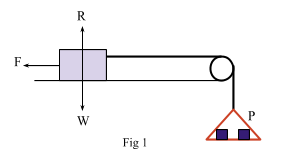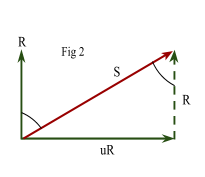# The Coefficient of Friction

Contents

## Summary

• Restriction to a motion is known as Frictional Force.
• Limiting Equilibrium is when frictional contact force is maximum, which happens when the object is at the point of sliding or moving.
• The magnitude of the limiting friction bears a constant ratio$\mu$,  to the normal contact force R.
• For General equilibrium$F\quad \le \quad \mu R$
• For Limiting equilibrium$F\quad \ =\quad \mu R$
•$\mu$  (known as mew) is called the coefficient of friction
• Angle of friction:$tan\quad \lambda \quad =\quad \mu$

Any restriction to a motion is known as Frictional Force. This force is unavoidable, until now we have been ignoring the effect of frictional force on any moving object but now in this article we will consider the effect of this force and introduce a new coefficient of friction.

#### Concept of Frictional Contact Force

1.    When an object is in contact with another body and is in equilibrium, then the frictional contact force is just sufficient to maintain this equilibrium. By applying a force on this object the frictional force increases along with the force to prevent the motion.
2.    When the same object is at the point of sliding or moving, the frictional contact force is maximum here. This state is known as ”Limiting Equilibrium”.
3.    At the point where the object starts moving, the frictional contact force is slightly less then from when the object was is limiting equilibrium. We don’t consider this slight difference in the forces in our calculations as it is very small difference.

We must remember a few important laws of friction:

• Direction of the frictional force is always opposite to the direction in which the body is moving.
• The limiting friction does not depend on the extent and shape of the surface in contact.
• When a body starts moving, the friction still opposes the motion, but it is slightly less than the limiting friction.
• When a body is at the point of moving, the equilibrium is said to be limiting. The magnitude of the limiting friction bears a constant ratio$\mu$,  to the normal contact force R. The ratio depends upon the nature of the surface and$F\quad =\quad \mu R$.

#### Coefficient of Friction

Let’s move on to study what the coefficient of friction is. Let’s suppose a block is lying on a rough table. It is connected to a light pan P with a light inextensible string as shown in Fig 1.When we put different weights in the pan, the block does not move. This shows that Frictional Force is equal to the weights in the pan P. We keep adding weights to the pan until the stage where the block is about to move. Now the frictional contact force opposing this motion is maximum. Through several experiments conducted, it is found that the frictional contact force bears a constant ratio to the normal contact force and this constant depends upon the roughness of the two surfaces in contact.

Let:

R = Normal Contact Force
F = Frictional Contact Force

Then in limiting equilibrium:$\frac { F }{ R } \quad =\quad \mu \quad (constant)$$\mu$  (known as mew) is called the coefficient of friction and thus maximum value of the frictional contact force is defined as:$F\quad =\quad \mu R$

Remember, F is not always equal to$\mu R$.  For General Equilibrium it may have value from 0 up to$\mu R$. For General equilibrium$F\quad \le \quad \mu R$.

#### Angle of frictionWhen the equilibrium is limiting, the angle$\lambda$  between the Normal contact force R and the total contact force S as shown in Fig 2 is known as the angle of friction:$tan\quad \lambda \quad =\quad \mu$

#### Example #1

Q. A particle of mass 2 Kg rests on a rough horizontal ground. The coefficient of friction between the particle and the ground is$\frac { 3 }{ 4 }$.  What force is required to make the particle move when pulling at an angle 45° to the horizontal.

Solution:

Let’s draw all the forces acting on the particle:Resolving horizontally

P cos 45=$\mu$ R

P (0.707) = 0.75 R

R = 0.943 P

Resolving vertically

R + P sin (45) = 20

0.943 P + 0.707P = 20

P = 12.1 N